# Intermediate Maths Solutions for 1A Exercise 1(a) Functions

Inter Maths 1A Functions Exercise 1(a) text book solutions are given.

These solutions are very easy to understand. Please study the text book lesson very well.

Observe the example problems and solutions. Try them again.

Observe the given solutions and try them in your own method.

You can see the text book solutions for

Inter Maths 1A textbook solutions

Inter Maths 1B textbook solutions

Inter Maths IIA textbook solutions

Inter Maths IIB textbook solutions

Functions

Exercise 1(a)

Exercise 1(b)

Exercise 1(c)

M

## Inter Maths Solutions for 1A Exercise 1(a) Functions

Exercise 1(a)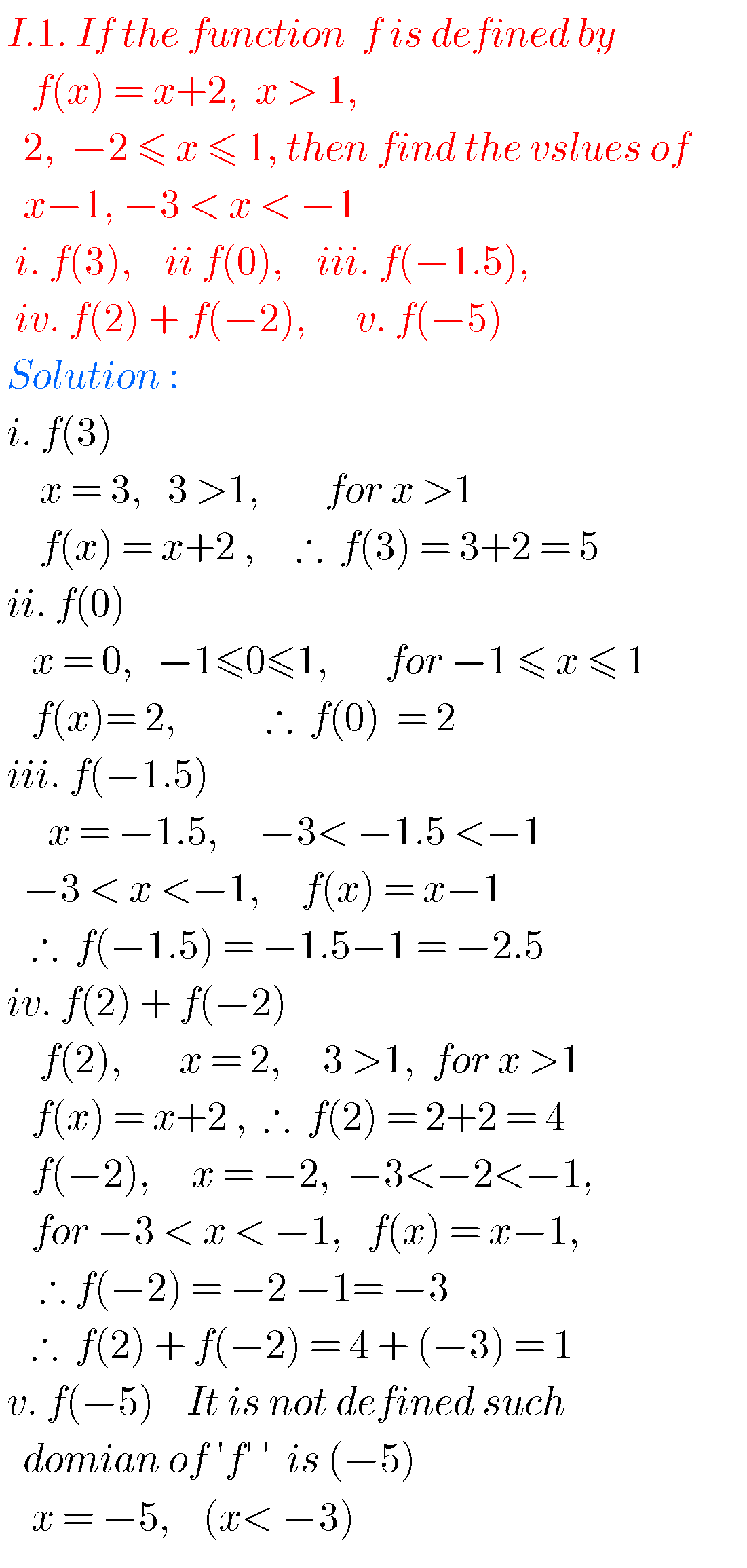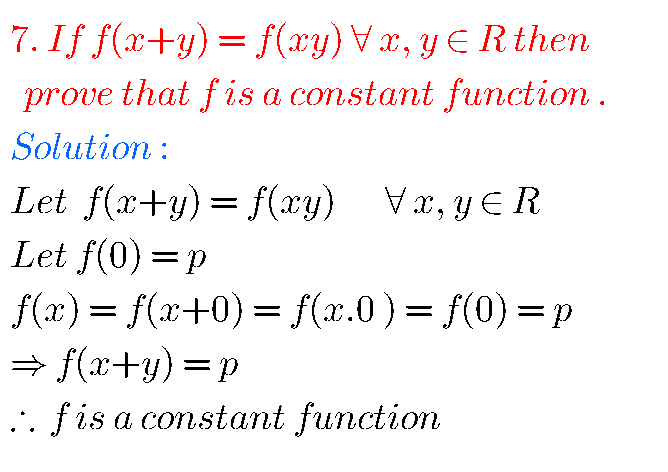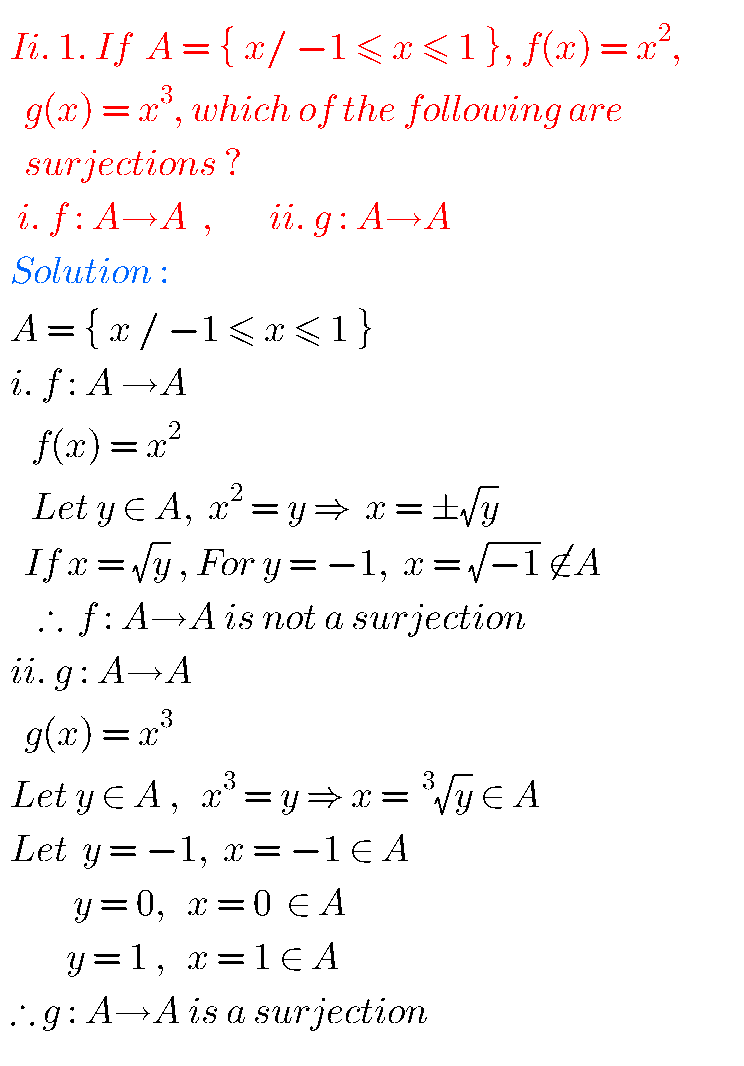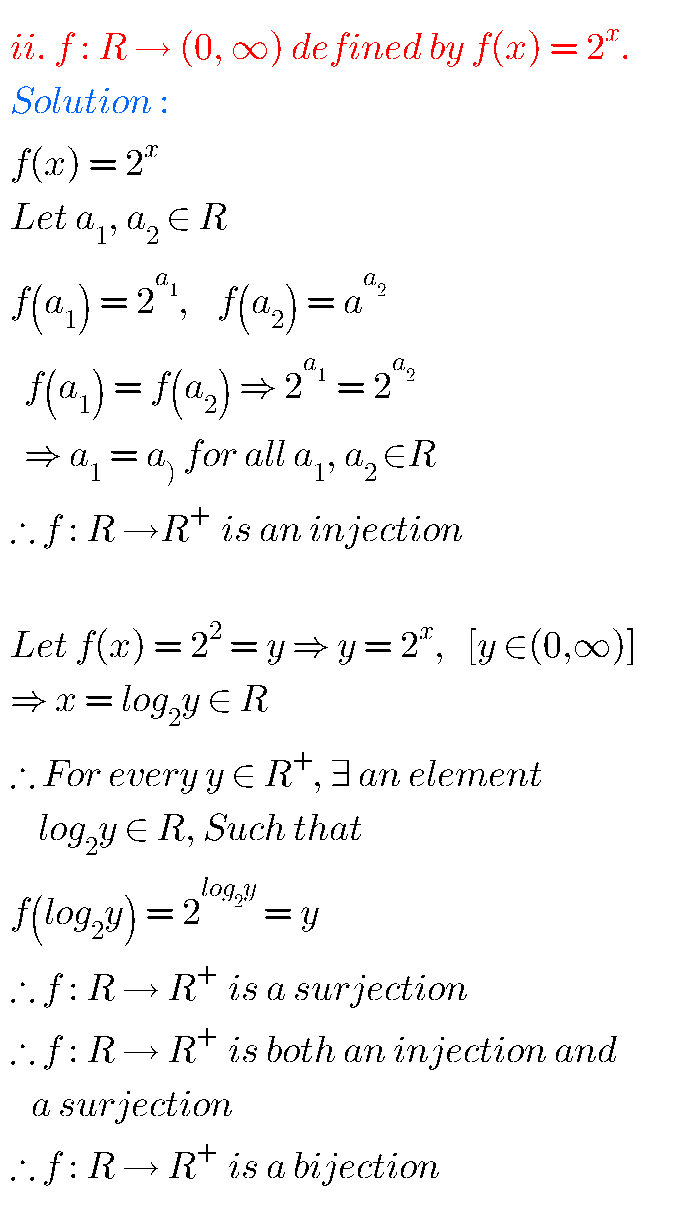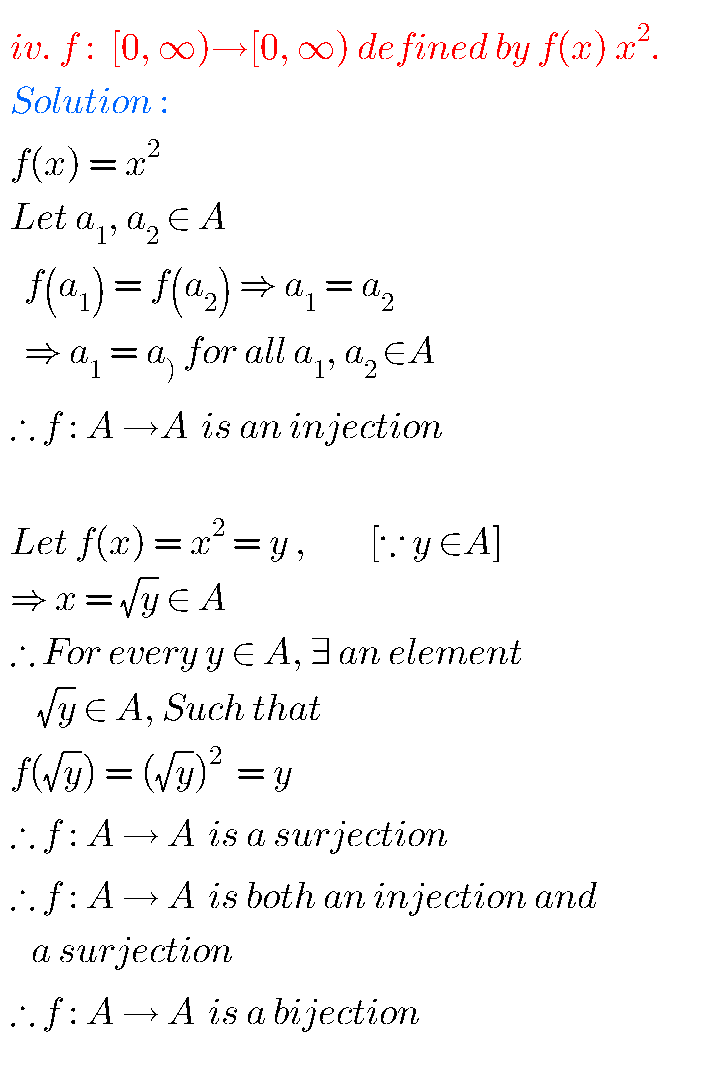## Functions Solutions Exercise 1(a) Inter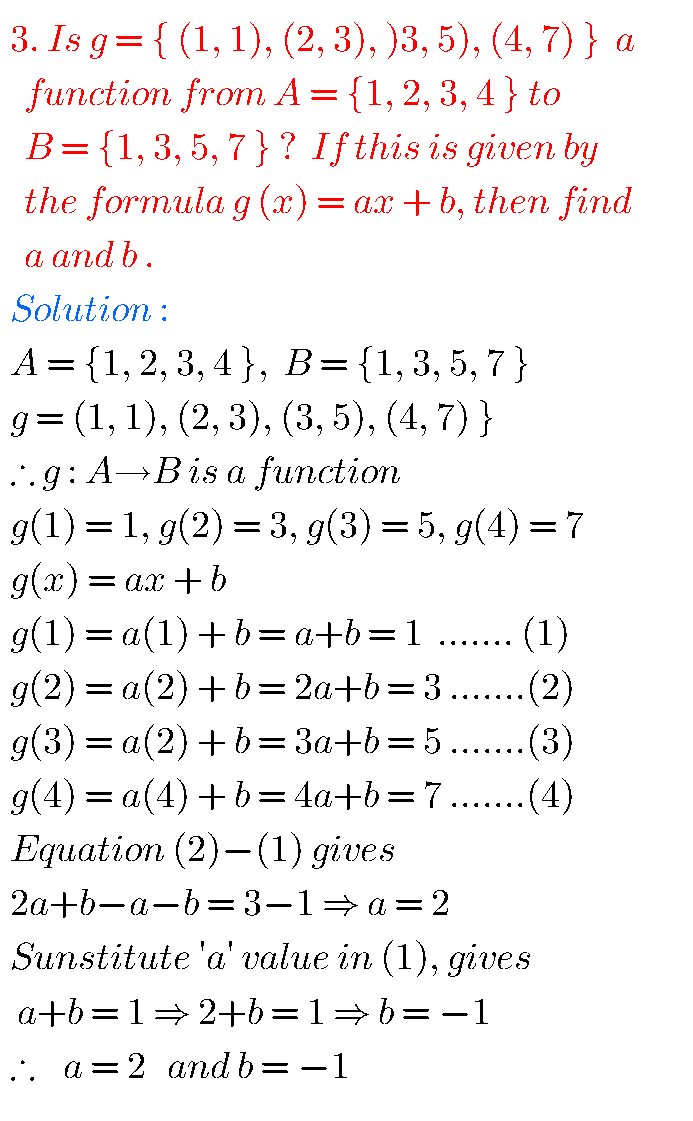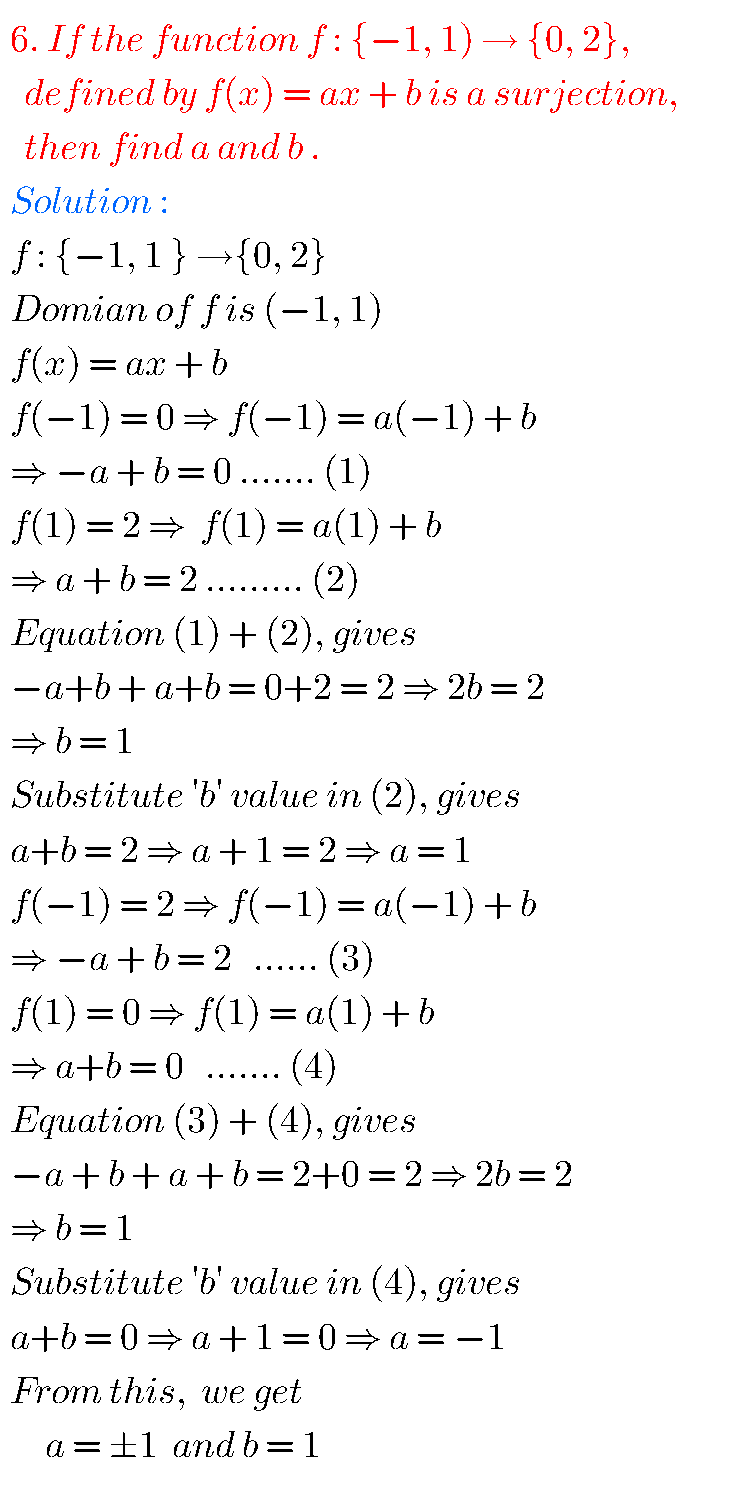Note : Observe the solutions and try them in your own method.

Some more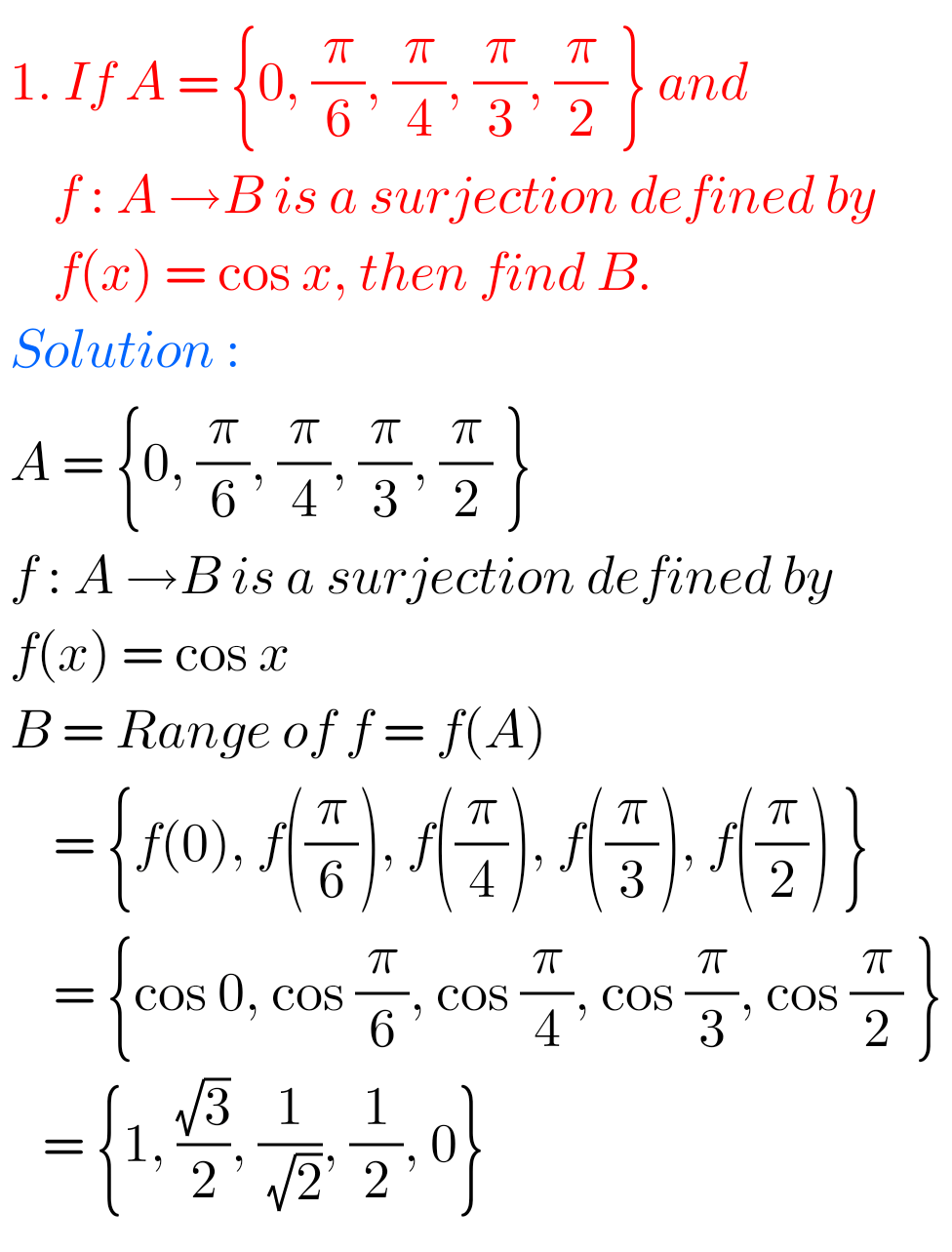SSC Maths solutions class 10

Ncert maths class 6 chapter 14 solutions

Nios maths 311 book 1 Sets 1.1 solutions

### 2 thoughts on “Intermediate Maths solutions for 1A Exercise 1(a) Functions”

1.It is super to study the simple problems and it’s also better to get full marks in final examination

2.This notes is very useful